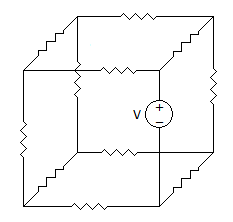# Electronics and Communication Engineering - Exam Questions Papers - Discussion

Discussion Forum : Exam Questions Papers - Exam Paper 1 (Q.No. 1)
1.
In the following non planar graph number of independent loop equations are8
12
7
5
Explanation:

Number of independent loop equations are given by,

L = B - N + 1

where L : No. of loop equations

B : No. of branches = 12

N : No. of nodes = 8

L = 12 - 8 + 1 = 5.

Discussion:
12 comments Page 1 of 2.

K Nireekshan said:   2 years ago
Loop =number of branches - number of nodes +1.

* branch means individual circuit line (having capacitor or resister or inductor) in it.
* node means junction of three branches at a point is known as a node.

In the above-given figure there are 11 branches are there and 7 nodes are there so for we know the formula for "loop" Is L=B-n+1.
So, L=11-7+1,
L=4+1,
L=5.

Sandy said:   5 years ago
Can someone please explain it in detail?
(1)

MOHAN said:   6 years ago
No of lines connected between 2 points or nodes having minimum of one element (r, l, c).

Nikhil kumar said:   6 years ago
How the number of branches is 12, can anybody explain?

Lakshmi durga said:   6 years ago
Planar graph that can be drawn without edges that intersects within a plane.

Non planar graph that cannot be drawn without edges that intersects within a plane.

Achu said:   6 years ago
Can anyone explain what is meant by planar and non planar graph?

Jayasudha said:   8 years ago
This answer explanation is good and also say some different concept.

Amarjeet said:   8 years ago
Node:

A point or junction where two or more circuit's elements (resistor, capacitor, inductor etc) meet is called Node.

Branch:

That part or section of circuit which locate between two junctions is called branch. In branch, one or more elements can be connected and they have two terminals.

Loop:

A closed path in circuit where more than two meshes can be occurred is called loop i.e. there may be many meshes in a loop, but a mesh does not contain on one loop.

Mesh:

A closed loop which contains no other loop within it or a path which does not contain on other paths is called Mesh.

Saravana said:   8 years ago
I don't know which is node and branches so explain thus concept.

JITEN said:   9 years ago